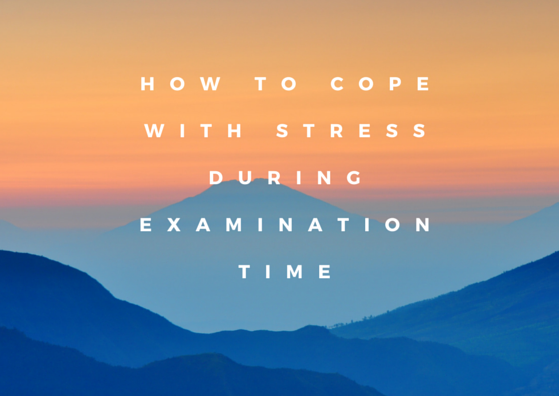Home » Tips and tricks » Page 3

# Tips and tricks

Tips and tricks are important for getting good scores, passing the competitive examinations. It helps us cut time in solving the problem.  This category list various tips and tricks on maths and physics which will be very helpful to the student.

Tips and tricks articles can be used from class 9 to competitive examination

## How to find acceleration with velocity and distance

In this article learn how to find acceleration with velocity and distance. We will look at the cases for motion with uniform acceleration and non-uniform acceleration in one dimension. We know that acceleration is defined as a change in velocity per unit time. So given velocities and time, we can calculate acceleration. Now here we …

## how to find acceleration with velocity and time

Acceleration is defined as change in velocity per unit time. It is a vector quantity and Average acceleration formula is defined as $a = \frac {Final \; velocity – Initial \; velocity}{ time \; taken }$ We will look at steps on how to find acceleration with velocity and time in various situation Uniformly Acceleration Motion …

## Conservative and Non conservative forces

Some Points about Conservative forces and Non conservative forces
1) Potential energy are defined for Conservative forces
2) An object that starts at a given point and return to the same point,then
net workdone by the conservative force is zero while in case of non conservative force ,it will not be zero

## Thermodynamics Problem solving tips

Net Heat flow at a junction is Zero
Internal Energy does not depend on the path
Internal Energy in a cyclic Process is zero
Workdone is given by the area enclosed by the process on the PV diagram
Always make sure you Heat(Q) ,Internal Energy(U) and Work(W) with correct sign in equation

## How to apply law of conservation of energy in mechanics

We often comes across the problems in mechanics where we need to apply the law of conservation of energy where gravitational potential energy or gravity is involved . For solving such problems you can consider the following problem solving strategy,

## Techniques for multiple choice questions for Examination

1) Read the instruction of the paper and see if there is any negative marking. This will help you in making educated guess.There are two types of Multiple choice questions. a) Only one answer b) can be more than one. Always check the instruction before attempting the question

2) Take a brief tour of the paper,so that you have a idea of the whole paper. Start your paper with Easy question.

3) Read the question before you look at the answer. Come up with the answer in your head before looking at the possible answers, this way the choices given on the test won’t throw you off or trick you.

4) Cross out any answers that you know are wrong.## 11 Smart ways to relieve stress during examination time

Students deal with lots of stress during the examination days. We can reduce the stress and stay calm and get success by following these simple steps

1)Take practice tests. The single best way to prepare is to practice. Taking sample exams under similar conditions as the real one will familiarize you and increase your confidence Level. This should be done few days before the examination days. The day before examination should be kept for revising the notes only

## Problem solving tricks-Right hand rules (Magnetic fields due to currents)

Right hand rule for cross product:-
Consider that c is the cross product of two vectors a and b i.e.,
c=a x b
If direction of vector a is along the x axis and that of b along z axis then direction of vector c could be find using right hand rule for cross products.